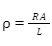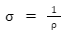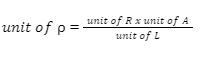• Call Now

1800-102-2727•

# Unit of Specific Resistance

A material's resistivity or specific resistance is a measure of the resistance it provides to the flow of electricity through it. It is a material's inherent quality. Specific resistance is determined by the material's composition, pressure, and temperature. Specific conductance, or the capacity to conduct electricity, is defined as the reciprocal of resistivity. The resistivity of conductors is quite low, whereas that of insulators is very high. A material's resistivity is a scalar quantity. Specific resistance, like every other physical property, requires a number linked with a unit.

## Resistivity is defined as

A conductor's resistance R is determined by its length L, cross-section A, and composition. The resistance of a conductor with a constant cross-section is proportional to its length. For a certain length, the resistance is inversely proportional to the cross-section. These two dependencies may be written as a pair as,
R ∝ L/A
R = L/A

The particular resistance is a proportionality constant in this case. For various materials, the constant has varying values. Specific resistance is determined by the material's physical qualities, such as density and composition. For unit length and unit cross-sectional area, L=1 and A=1, respectively.
= R

Resistivity may be defined using this condition.
The resistivity or specific resistance of a material is defined as the resistance of a homogenous chunk of that material of unit length and unit cross-section. Quantitatively,Material conductivity is defined as the inverse of resistance.## Resistivity Unit

According to the particular resistance formula,When resistance R is stated in Ohms () and distances are expressed in centimetres, one usable unit is produced (cm). The unit of resistivity in this system is Ohmcm (.cm).

When lengths are measured in metres (m), the SI unit of specific resistance is Ohmm (.m). Ohm-1 m-1 or siemens m-1 is the SI unit of conductivity (S . m-1).
Metals conduct electricity well, but insulators can transport little to no current.

Metals have a relatively low specific resistance (10-8.m), whereas common insulators have quite high values (1016.m). The resistivity values at 20 degrees Celsius for various common materials are shown below.

## Different Materials' Specific Resistance

 Conductors Materials Resistivity (m) Platinum 1.06 x 10-7 Zinc 5.90 x 10-8 Tin 1.09 x 10-8 Silver 1.59 x 10-8 Copper 1.68 x 10-8 Iron 9.70 x 10-8 Gold 2.44 x 10-8 Insulators Rubber 1013 Diamond 1012 Glass 1011 - 1015 Air 109 - 1015

## Copper Resistivity

Copper has a specific resistance of 1.68 x 10-8. m (20 degree celsius), which means that the resistance between two opposed sides of a copper cube of 1 m is 1.68 x 10-8 at temperature 20 degree celsius. Copper has a conductivity of 5.96 x 107 Sm-1. Copper's resistance to current flow is minimal due to its extremely low resistivity and high conductivity. Copper wires are commonly used to carry electricity in electrical circuits.

## What Did You Know?

• The specific resistance varies with the ambient temperature. For metals, it rises as temperature rises. The resistivity of glass, on the other hand, falls significantly at very high temperatures.
• In the superconducting state, superconductors have zero resistance (at very low temperature).
• Semiconductors' resistance reduces as temperature rises.Talk to our expert
Resend OTP Timer =
By submitting up, I agree to receive all the Whatsapp communication on my registered number and Aakash terms and conditions and privacy policy